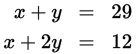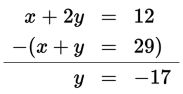# SAT Math Multiple Choice Question 796: Answer and Explanation

### Test Information

Question: 7961. If the ordered pair (x, y) is the solution to the system of equations above, what is the value of y?

• A. –17
• B. 12
• C. 46
• D. 75

Explanation:

A

Difficulty: Easy

Category: Heart of Algebra / Systems of Linear Equations

Strategic Advice: When solving a system of linear equations, always look at the coefficients of the variables to decide on a strategy. Here, because you're looking for the value of y, subtracting the first equation from the second equation will get you to the answer the quickest (because the x's will be eliminated and you'll be left with y).

Getting to the Answer: Rewrite the equations with the second equation on top. Then, carefully subtract: